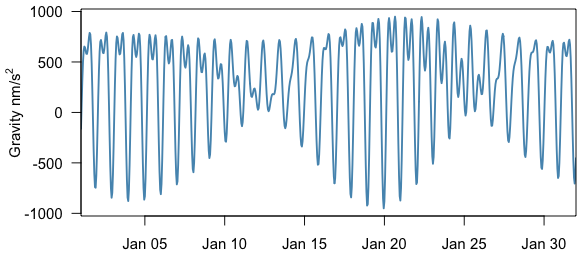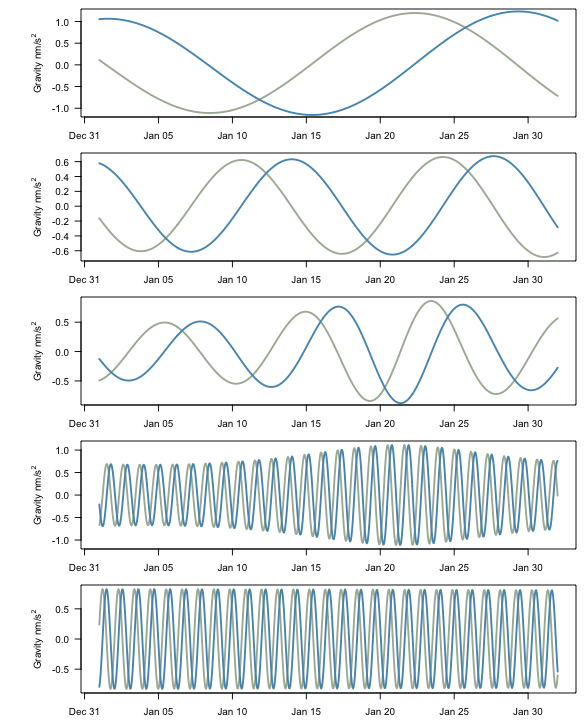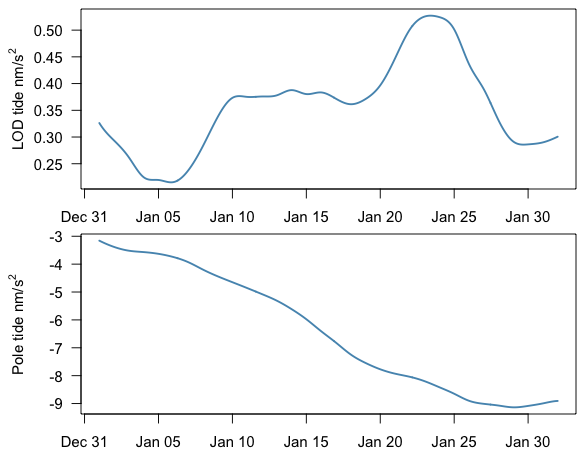# Introduction to the earthtide package

## Code background

earthtide is a port of the ‘Fortran ETERNA 3.4’ (Wenzel, 1996) predict and part of the analyze codes with the Kudryavtsev 2004 update. The original ‘Fortran’ code was rewritten in R, and C++ using Rcpp, RcppArmadillo, and RcppParallel. The package is useful for generating synthetic earth tides using highly accurate tidal catalogs for prediction and regression. Attempts were made to ensure that results were consistent with the ‘ETERNA 3.4’, however, there is always the possibility that a bug was introduced during the conversion and update.

## Capabilities

The following tidal componenents are implemented in earthtide.

Tidal component Status Output units
tidal_potential tested $$meters^2/second^2$$
gravity tested $$nanometers/second^2$$
tidal_tilt tested $$milliarcsec$$
vertical_displacement tested $$millimeter$$
horizontal_displacement preliminary $$millimeter$$
n_s_displacement preliminary $$millimeter$$
e_w_displacement preliminary $$millimeter$$
vertical_strain tested $$nanostrain$$
areal_strain tested $$nanostrain$$
volume_strain tested $$nanostrain$$
horizontal_strain tested $$nanostrain$$
ocean_tides preliminary $$millimeter$$

## Example

The primary inputs are the date-time in UTC, the component name from the previous table, and the latitude and longitude. For most cases these are the minimum requirements necessary. For the full list of options see the documentation for calc_earthtide.

tms <- as.POSIXct("2015-01-01", tz = "UTC") + 0:(24*31) * 3600

gravity_tide <- calc_earthtide(utc = tms,
method = 'gravity',
latitude = 52.3868,
longitude = 9.7144)

There are two main methods of generating Earth tides: predict and analyze. Predict returns the combined tidal signal, and analyze returns a set of sin and cos curves for each wave group that is specified. This option is set using the do_predict parameter which defaults to TRUE.

### Predict

gravity_tide <- calc_earthtide(utc = tms,
do_predict = TRUE,
method = 'gravity',
latitude = 52.3868,
longitude = 9.7144)### Analyze

In analyze mode, results are separated by wave group into sin and cos curves. The resulting sin and cos curves can be used in further analysis such as least squares models. The first five constituents are plotted in the following example. The wave_groups parameter is specified using a data.frame having the start and end frequencies for each component.

wg <- eterna_wavegroups
wg <- na.omit(wg[wg$time=='1 month',]) tides <- calc_earthtide(utc = tms, do_predict = FALSE, method = 'gravity', latitude = 52.3868, longitude = 9.7144, wave_groups = wg)## Wave grouping The choice of wave groups is important. Wave groups are specified using a data.frame of start and end values for each group. Example groupings are provided in the dataset eterna_wavegroups. The choice of the appropriate wave groups is dependent purpose of the study and the duration of the dataset to be analyzed. For example, if the goal is to generate tidal harmonics to analyze one (1) month of data you would select the “1 month” dataset. tms <- as.POSIXct("2015-01-01", tz = "UTC") + 0:(24*31) * 3600 wg <- eterna_wavegroups wg <- na.omit(wg[wg$time=='1 month',])

head(wg)
##   name    start      end    time
## 3   MM 0.020885 0.054747 1 month
## 4   MF 0.054748 0.091348 1 month
## 5  MTM 0.091349 0.501369 1 month
## 6   Q1 0.501370 0.911390 1 month
## 7   O1 0.911391 0.947991 1 month
## 8   M1 0.947992 0.981854 1 month

## LOD (length of day) tide and pole tide

The Length of Day (LOD) and Pole tides can also be calculated. These results differ from ETERNA in that we interpolate using splines.

tide <- calc_earthtide(utc = tms,
method = c('lod_tide', 'pole_tide'),
latitude = 52.3868,
longitude = 9.7144)## Speed for large datasets

There are two primary ways to increase the speed of generating the tidal datasets: increasing the astro_update parameter; and increasing the cutoff parameter. In general, the speed should be as good as or better than the fortran version of ETERNA, given the parallel computation. The cutoff parameter determines the number of waves used in the analysis. A larger cutoff value means fewer waves will be used leading to a faster but less accurate result. The astro_update parameter determines how often the astronomical arguments are updated. In ETERNA this value ranges from daily to yearly. The current default in the earthtide package for astro_update is 1, which means that the parameters are updated for every time provided, and therefore if you are predicting in hourly increments it will be updated 24 times a day. Increasing this value typically speeds up computation, and in general values larger than 1 can be safely used unless the time interval between predictions is large.

## TODO:

This package is still in development. The following changes are planned:

• Speed enhancements
• Print methods and getter/setters for love numbers, catalog, station variables
• Optional wave group names